# Mildly Amusing Statistical Card Game: Example Hand

You are here: Home > Maths intro > MASC 2

# HomeCard GameExample Hand

Hand 1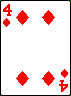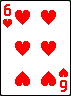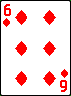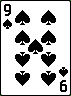Scoring

Range:minusgives 7

Mode:so mode is 6

Median:is the middle card,so score is 6

Mean:

2 + 4 + 6 + 6 + 9 = 27
27/5 = 5.4, so score is 5.4

Total Score for Hand 1:

7 + 6 + 6 + 5.4 = 24.4 so score is 24.4

Hand 2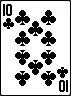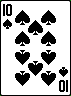Scoring

Range:minusgives 9

Mode:so mode is 10

Median:is the middle card,so score is 7

Mean:

1 + 5 + 7 + 10 + 10 = 33
33/5 = 5.4, so score is 6.6

Total Score for Hand 2:

9 + 10 + 7 + 6.6 = 32.6 so score is 32.6

Hand 1: 24.4; Hand 2: 32:6 so Hand 2 is the winner